# Persistent Structures in Rule 110

The known families of persistent structures in the Rule 110 elementary cellular automaton

Originator: Stephen Wolfram

These structures were given on pages 292 through 296 of Stephen Wolfram's "A New Kind Of Science".

This resource also contains specifications for the collisions between structures shown on pages 294 through 296.

## Examples

### Basic Examples

Retrieve the resource:

 In:=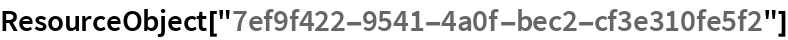Out=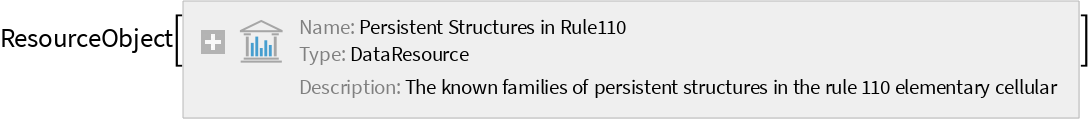Retrieve the default content:

 In:=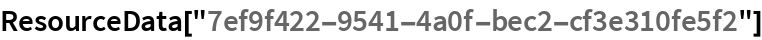Out=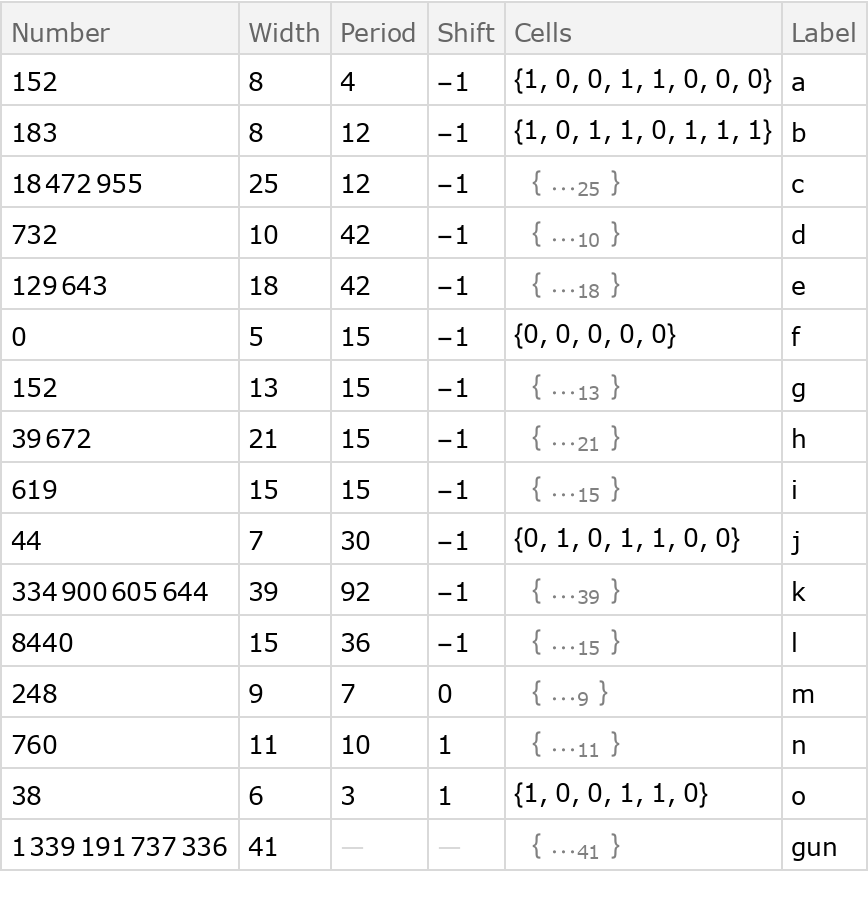Get the initial conditions for a block of “background” in the evolution:

 In:=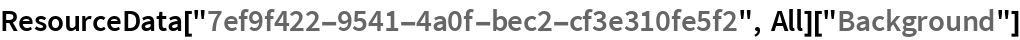Out=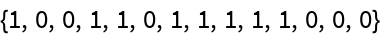Generate a sample of “background” evolution:

 In:=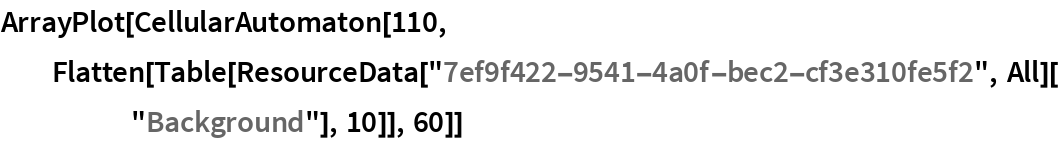Out=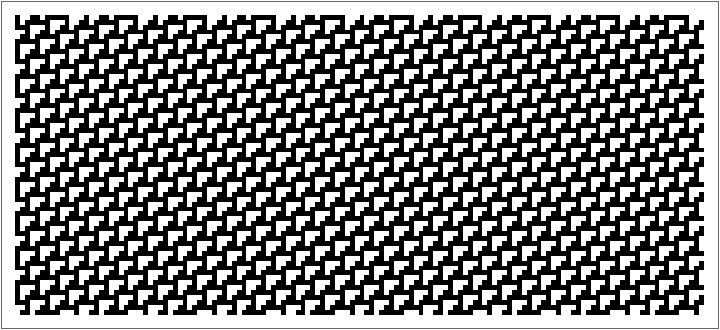Generate images of the evolution for the first 5 structures:

 In:=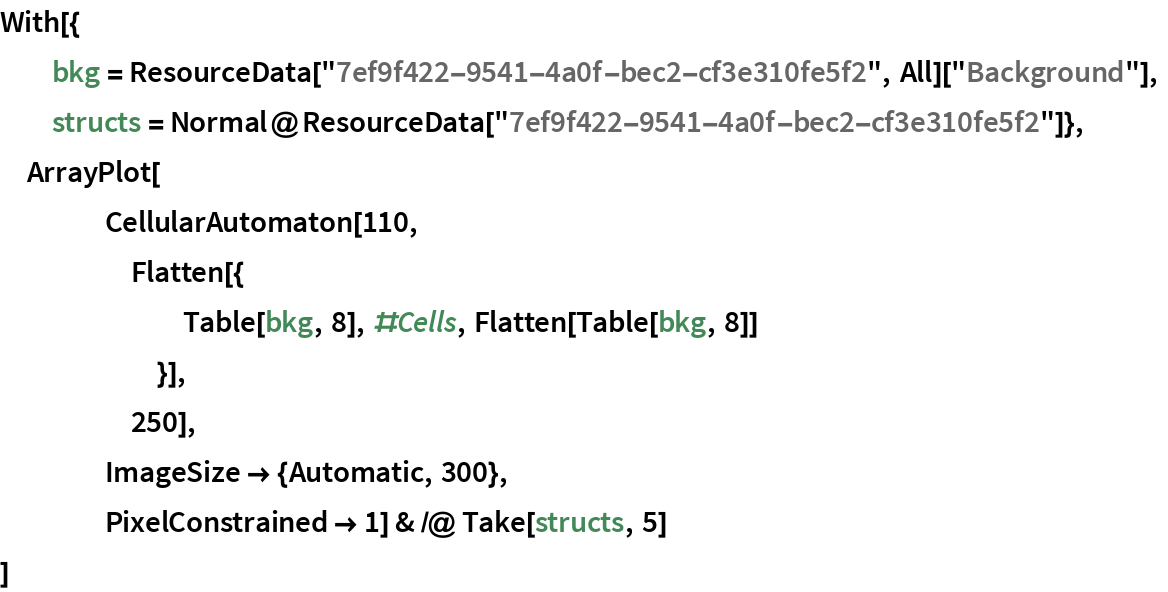Out=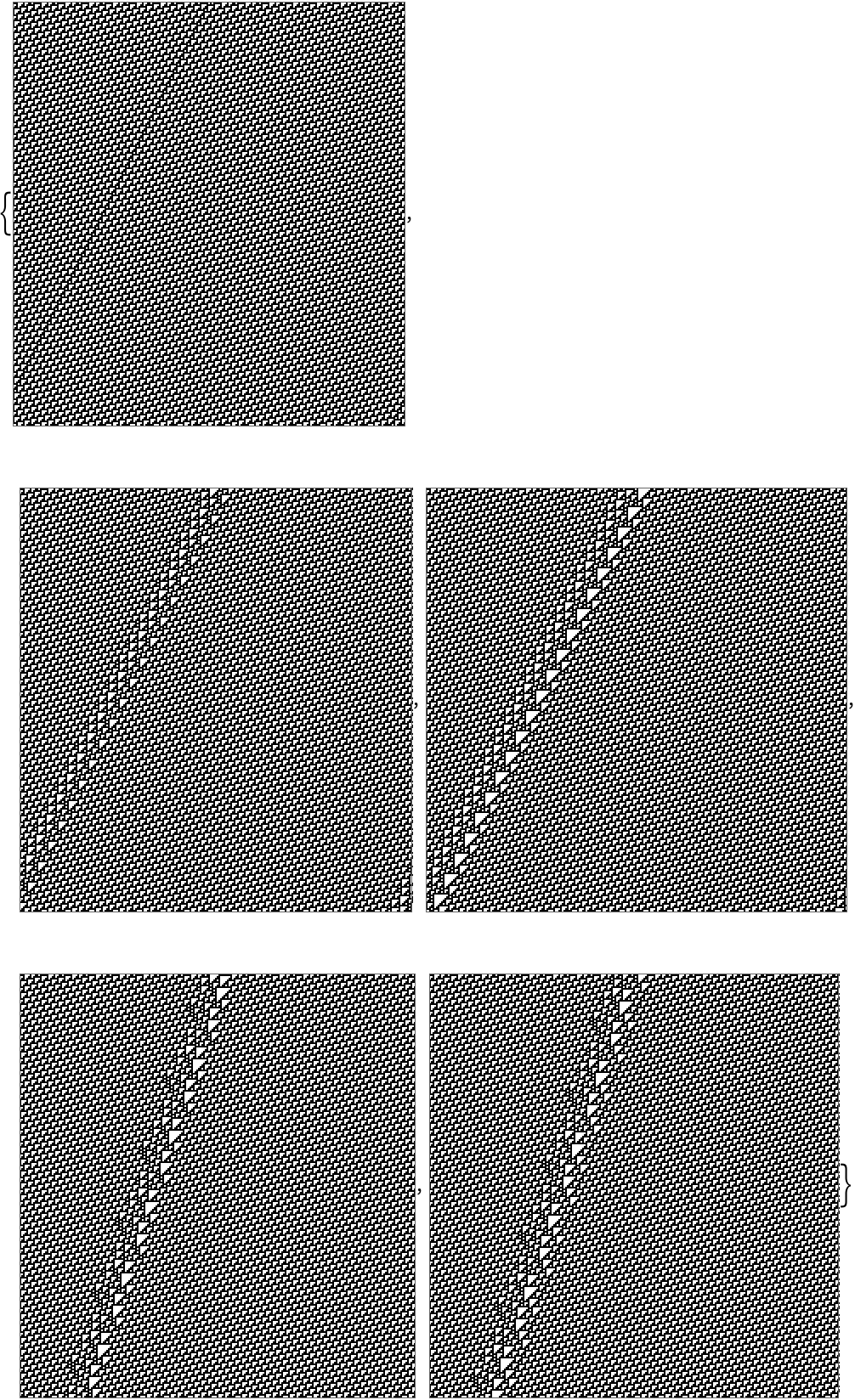Create a composite images similar to the one on page 292:

 In:=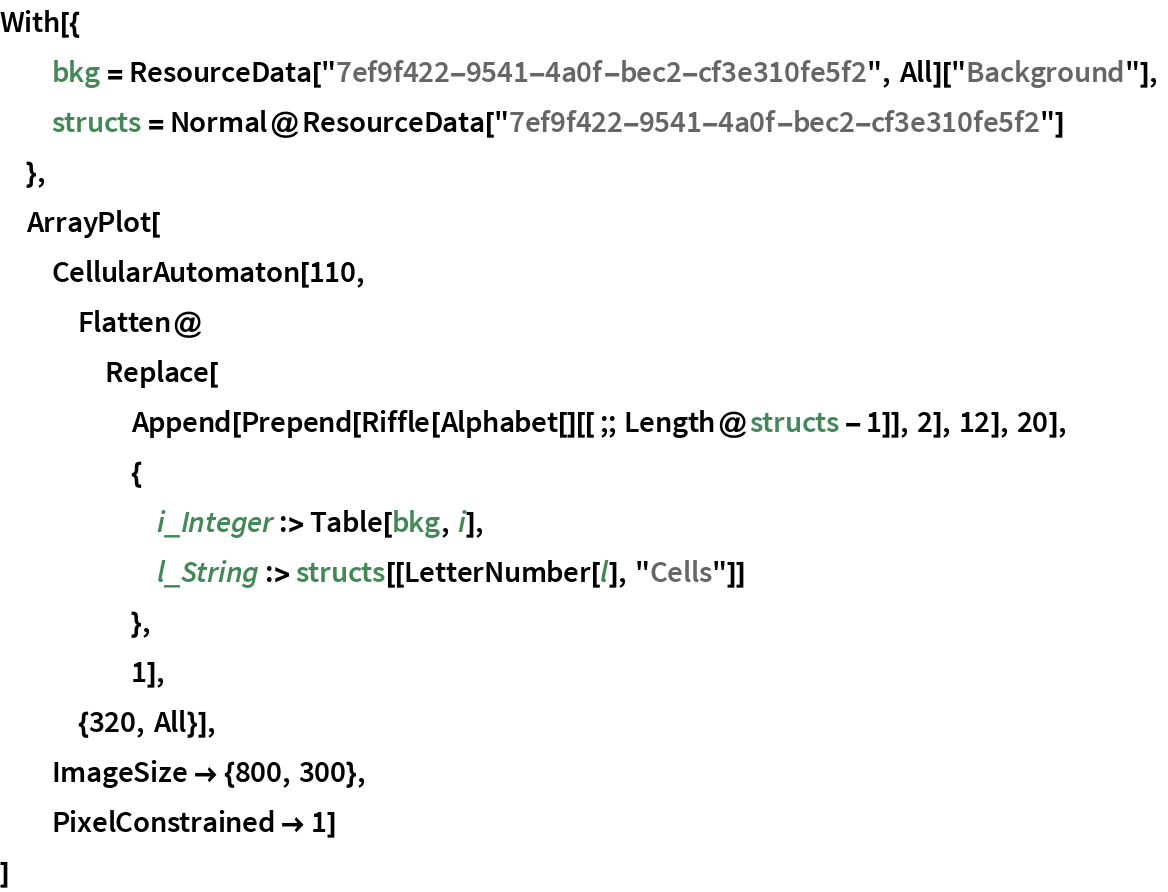Out=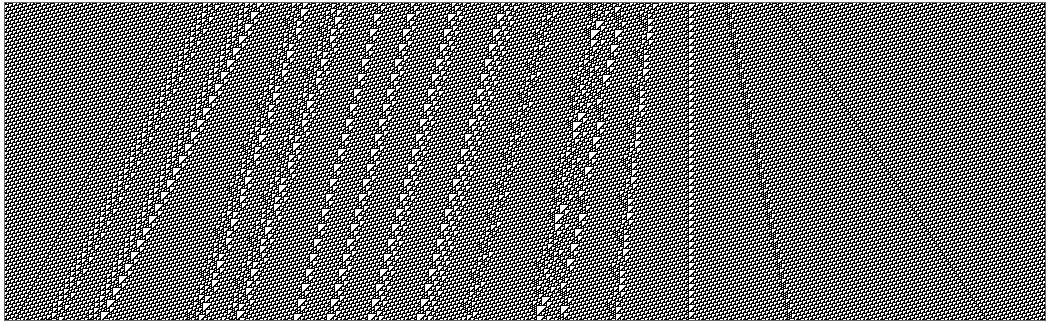Generate the images from page 294:

 In:=Out=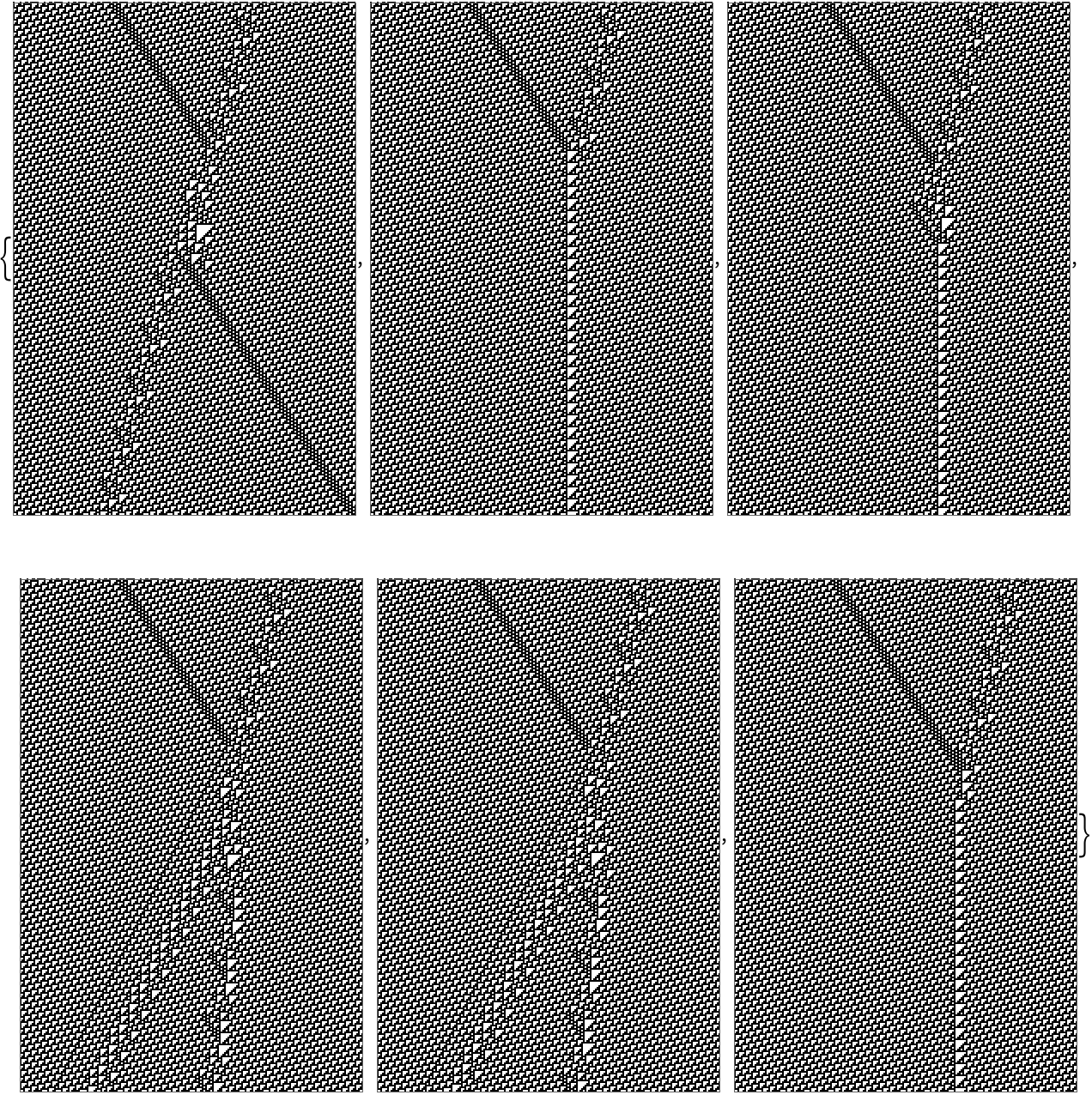In:=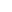Wolfram Research, "Persistent Structures in Rule 110" from the Wolfram Data Repository (2017) https://doi.org/10.24097/wolfram.75532.data